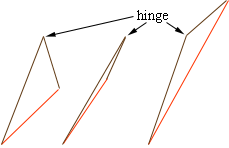Quandaries and Queries annette parent level 10-11       How do you find the length of a triangle side if you know two sides? Hi Annette, If you know the lengths of two sides of a triangle and you want to find the length of the third side you need some more information. Imagine that you have two sticks of the correct lengths to represent the two sides you know, and the two sticks are hinged together at one end. If you change the angle between the sticks (the amount the hinge is open) you can form triangles with the third side of different lengths.If you know the angle between the two known sides or if you know that the triangle is a right triangle (and you know which of the angles is a right angle) you can find the length of the third side. Penny Go to Math Central